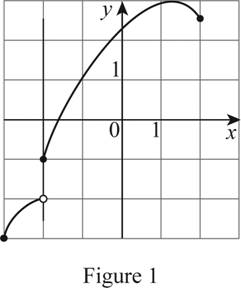# Whether the curve is the graph of a function of x . If so, find the domain and range of the function.### Single Variable Calculus: Concepts...

4th Edition
James Stewart
Publisher: Cengage Learning
ISBN: 9781337687805### Single Variable Calculus: Concepts...

4th Edition
James Stewart
Publisher: Cengage Learning
ISBN: 9781337687805

#### Solutions

Chapter 1.1, Problem 7E
To determine

## Whether the curve is the graph of a function of x. If so, find the domain and range of the function.

Expert Solution

The curve is the graph of a function of x.

The domain of the function is 3x2 and the range of the function is 3y<2 and 1y3.

### Explanation of Solution

Vertical line test states that the curve in the xy coordinate plane is the graph of a function of x if and only if a vertical line intersects the curve at exactly one point.

Perform the vertical line test for the given graph.

Draw a vertical line such that it passes through the curve as shown below in Figure 1.The given graph is a piecewise function, in which the open dot indicates that the point is excluded and the closed dot indicates that the point is included in the graph.

Moreover, it is observed from Figure 1 that the vertical line intersects the curve exactly at one point. Therefore, the curve is the graph of a function of x.

Since, the domain of a function is the set of all possible x values of the graph, the domain of the function is 3x2.

Since, the range of a function is the set of all possible y values of the graph, the range of the function is 3y<2 and 1y3.

### Have a homework question?

Subscribe to bartleby learn! Ask subject matter experts 30 homework questions each month. Plus, you’ll have access to millions of step-by-step textbook answers!